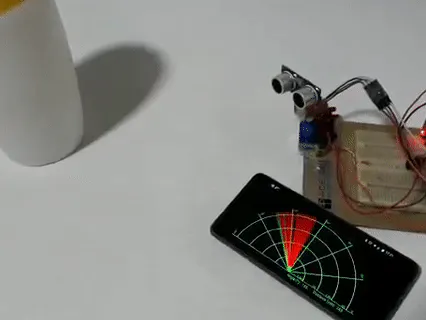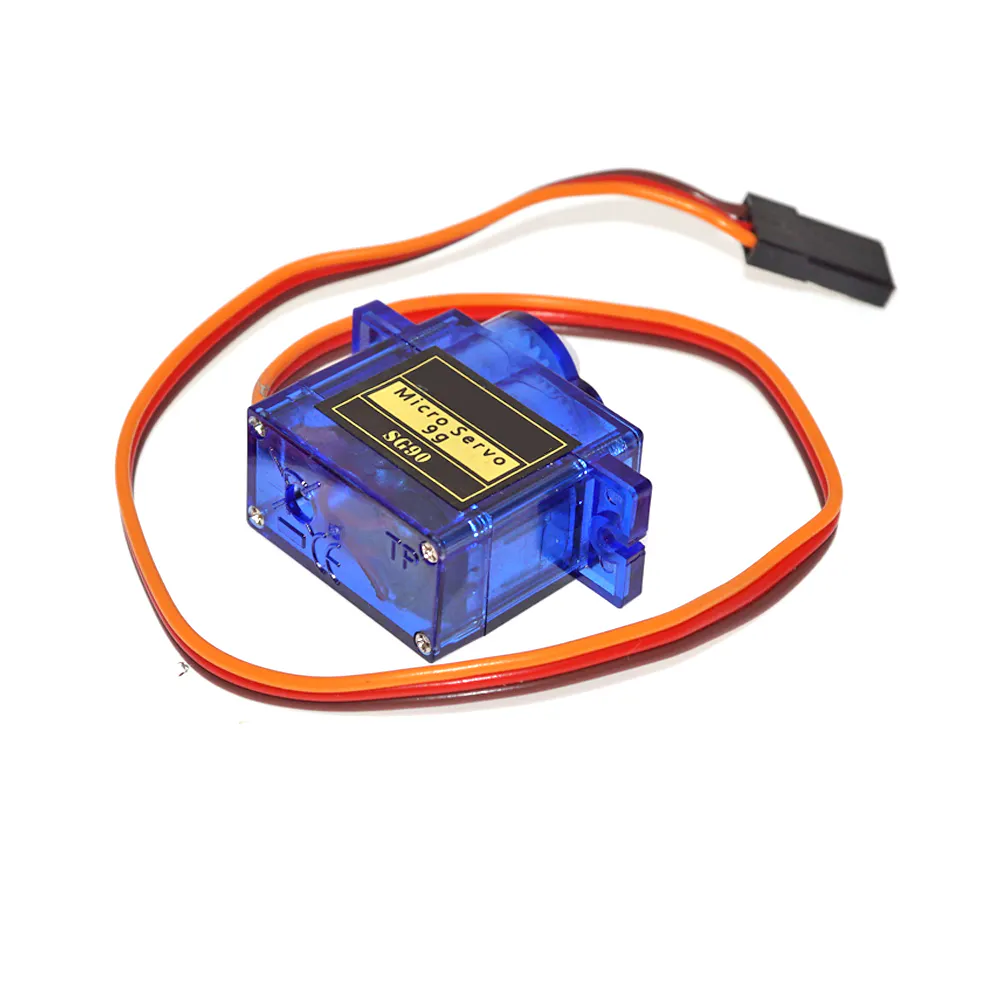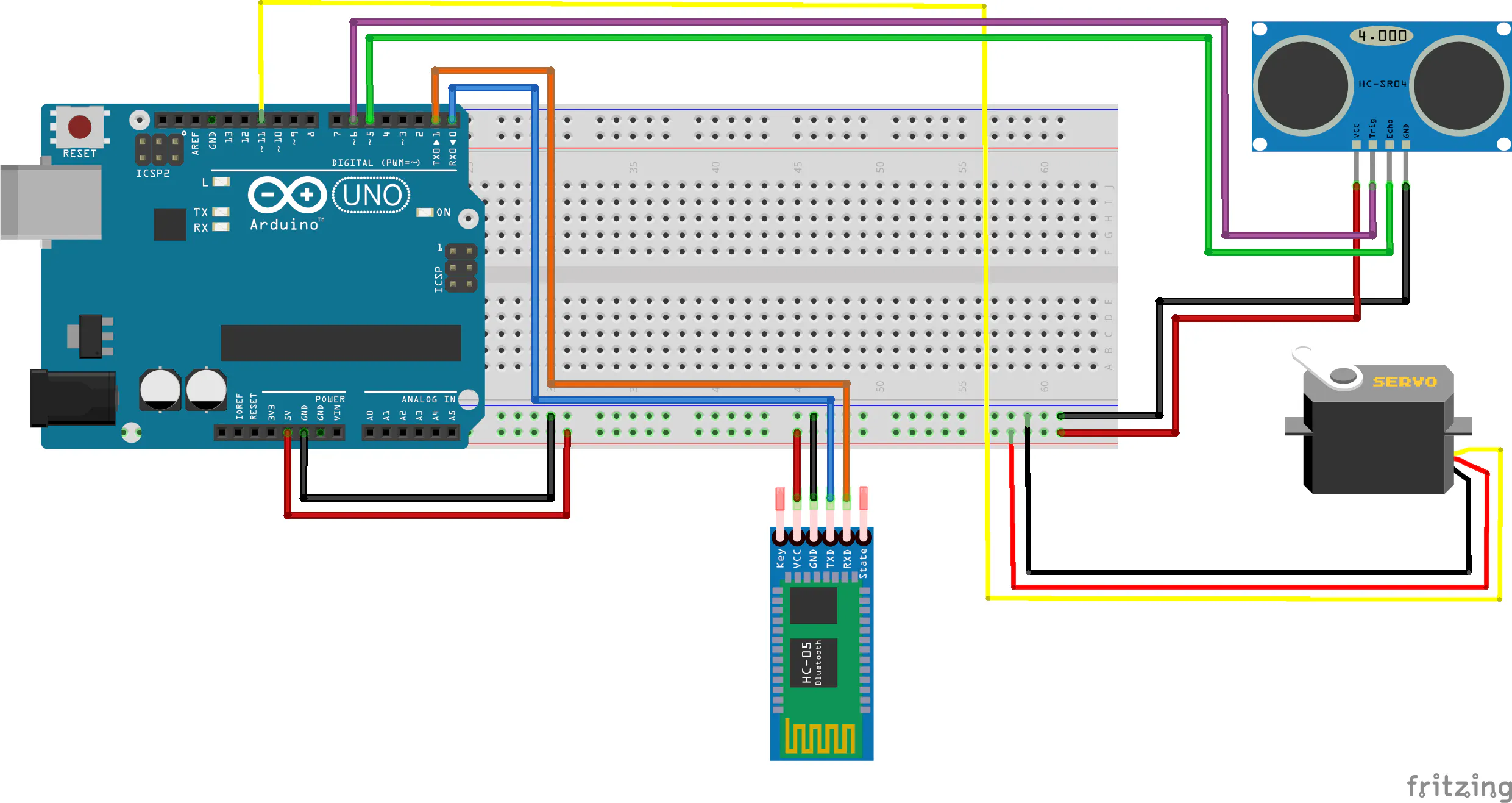# ArduSonar

Mini sonar made with Arduino and Android.

BeginnerShowcase (no instructions)2 hours277## Things used in this project

### Hardware componentsArduino UNO & Genuino UNO
×1SG90 Micro-servo motor
×1
 HC-06 Bluetooth Module
×1Ultrasonic Sensor - HC-SR04 (Generic)
×1

### Software apps and online servicesArduino IDEMIT App Inventor 2

## Schematics

### Connexions scheme## Code

### Arduino code

C/C++
```#include <Servo.h>

const int servoPin = 11;
const int trigPin = 6;
const int echoPin = 5;

int duration;
int distance;

Servo servo;

void setup() {
pinMode(trigPin, OUTPUT);
pinMode(echoPin, INPUT);

servo.attach(servoPin);

Serial.begin(9600);
}

void loop() {

for(int angle = 5; angle <= 175; ){
servo.write(angle);
delay(25);
distance = calculateDistance();
printData(angle, distance);
angle++;
}
}

for(int angle = 175; angle >= 5; ){
servo.write(angle);
delay(25);
distance = calculateDistance();
printData(angle, distance);
angle--;
}
}

}

// Calculate the distance to the nearest object
int calculateDistance(){
digitalWrite(trigPin, LOW);
delayMicroseconds(2);
digitalWrite(trigPin, HIGH);
delayMicroseconds(10);
digitalWrite(trigPin, LOW);
duration = pulseIn(echoPin, HIGH);
distance= duration*0.034/2;
return distance;
}

// Put the information in the serial port
int printData(int angle, int distance){
Serial.print(angle);
Serial.print(";");
Serial.println(distance);
}
```

### Github repository of project code

Here you could find the apk of the Android app made with app inventor.

## Credits

### Luis Cambero Piqueras

1 project • 0 followers